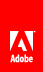# Groups

Flash Player 9 and later, Adobe AIR 1.0 and later

You can specify a group in a regular expression by using parentheses, as follows:

`/class-(\d*)/`

A group is a subsection of a pattern. You can use groups to do the following things:

• Apply a quantifier to more than one character.

• Delineate subpatterns to be applied with alternation (by using the | character).

• Capture substring matches (for example, by using \1 in a regular expression to match a previously matched group, or by using \$1 similarly in the replace() method of the String class).

The following sections provide details on these uses of groups.

#### Using groups with quantifiers

If you do not use a group, a quantifier applies to the character or character class that precedes it, as the following shows:

```var pattern:RegExp = /ab*/ ;
// matches the character a followed by
// zero or more occurrences of the character b

pattern = /a\d+/;
// matches the character a followed by
// one or more digits

pattern = /a{1,3}/;
// matches the character a followed by
// one to three occurrences of either 1, 2, or 3```

However, you can use a group to apply a quantifier to more than one character or character class:

```var pattern:RegExp = /(ab)*/;
// matches zero or more occurrences of the character a
// followed by the character b, such as ababab

pattern = /(a\d)+/;
// matches one or more occurrences of the character a followed by
// a digit, such as a1a5a8a3

pattern = /(spam ){1,3}/;
// matches 1 to 3 occurrences of the word spam followed by a space```

#### Using groups with the alternator (|) character

You can use groups to define the group of characters to which you want to apply an alternator ( | ) character, as follows:

```var pattern:RegExp = /cat|dog/;
// matches cat or dog

pattern = /ca(t|d)og/;

#### Using groups to capture substring matches

When you define a standard parenthetical group in a pattern, you can later refer to it in the regular expression. This is known as a backreference , and these sorts of groups are known as capturing groups . For example, in the following regular expression, the sequence \1 matches whatever substring matched the capturing parenthetical group:

```var pattern:RegExp = /(\d+)-by-\1/;
// matches the following: 48-by-48```

You can specify up to 99 of these backreferences in a regular expression by typing \1 , \2 , ... , \99 .

Similarly, in the replace() method of the String class, you can use \$1\$99 – to insert captured group substring matches in the replacement string:

```var pattern:RegExp = /Hi, (\w+)\./;
var str:String = "Hi, Bob.";
trace(str.replace(pattern, "\$1, hello."));
// output: Bob, hello.```

Also, if you use capturing groups, the exec() method of the RegExp class and the match() method of the String class return substrings that match the capturing groups:

```var pattern:RegExp = /(\w+)@(\w+).(\w+)/;
var str:String = "bob@example.com";
trace(pattern.exec(str));
// bob@example.com,bob,example,com```

#### Using noncapturing groups and lookahead groups

A noncapturing group is one that is used for grouping only; it is not “collected,” and it does not match numbered backreferences. Use (?: and ) to define noncapturing groups, as follows:

`var pattern = /(?:com|org|net);`

For example, note the difference between putting (com|org) in a capturing versus a noncapturing group (the exec() method lists capturing groups after the complete match):

```var pattern:RegExp = /(\w+)@(\w+).(com|org)/;
var str:String = "bob@example.com";
trace(pattern.exec(str));
// bob@example.com,bob,example,com

//noncapturing:
var pattern:RegExp = /(\w+)@(\w+).(?:com|org)/;
var str:String = "bob@example.com";
trace(pattern.exec(str));
// bob@example.com,bob,example```

A special type of noncapturing group is the lookahead group, of which there are two types: the positive lookahead group and the negative lookahead group.

Use (?= and ) to define a positive lookahead group, which specifies that the subpattern in the group must match at the position. However, the portion of the string that matches the positive lookahead group can match remaining patterns in the regular expression. For example, because (?=e) is a positive lookahead group in the following code, the character e that it matches can be matched by a subsequent part of the regular expression—in this case, the capturing group, \w*) :

```var pattern:RegExp = /sh(?=e)(\w*)/i;
var str:String = "Shelly sells seashells by the seashore";
trace(pattern.exec(str));
// Shelly,elly```

Use (?! and ) to define a negative lookahead group that specifies that the subpattern in the group must not match at the position. For example:

```var pattern:RegExp = /sh(?!e)(\w*)/i;
var str:String = "She sells seashells by the seashore";
trace(pattern.exec(str));
// shore,ore```

#### Using named groups

A named group is a type of group in a regular expression that is given a named identifier. Use (?P<name> and ) to define the named group. For example, the following regular expression includes a named group with the identifier named digits :

`var pattern = /[a-z]+(?P<digits>\d+)[a-z]+/;`

When you use the exec() method, a matching named group is added as a property of the result array:

```var myPattern:RegExp = /([a-z]+)(?P<digits>\d+)[a-z]+/;
var str:String = "a123bcd";
var result:Array = myPattern.exec(str);
trace(result.digits); // 123```

Here is another example, which uses two named groups, with the identifiers name and dom :

```var emailPattern:RegExp =
/(?P<name>(\w|[_.\-])+)@(?P<dom>((\w|-)+))+\.\w{2,4}+/;